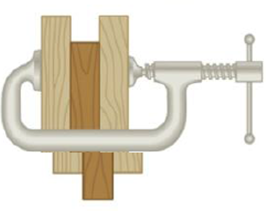Chapter 4, Problem 91AP

Chapter
Section
Textbook Problem

The board sandwiched between two other boards in Figure P4.91 weighs 95.5 N. If the coefficient of friction between the boards is 0.663, what must be the magnitude of the compression forces (assumed to be horizontal) acting on both sides of the center board to keep it from slipping?Figure P4.91

To determine
The magnitude of compression force.

Explanation

Given Info: Weight of the board is 95.5 N. The co-efficient of friction ( μs ) is 0.663.

The free body diagram is given below.

From the diagram,

2Fs=W (I)

• N is the compression force.
• W is the weight.
• Fs is the friction force.

The formula for the compression force is,

N=Fsμs

Still sussing out bartleby?

Check out a sample textbook solution.

See a sample solution

The Solution to Your Study Problems

Bartleby provides explanations to thousands of textbook problems written by our experts, many with advanced degrees!

Get Started